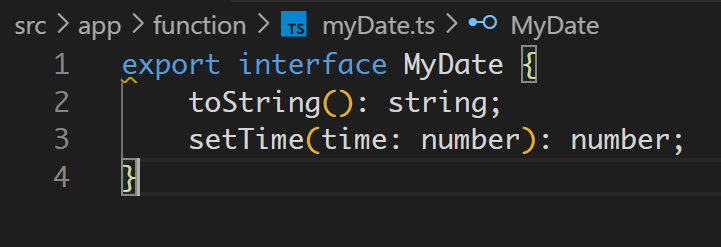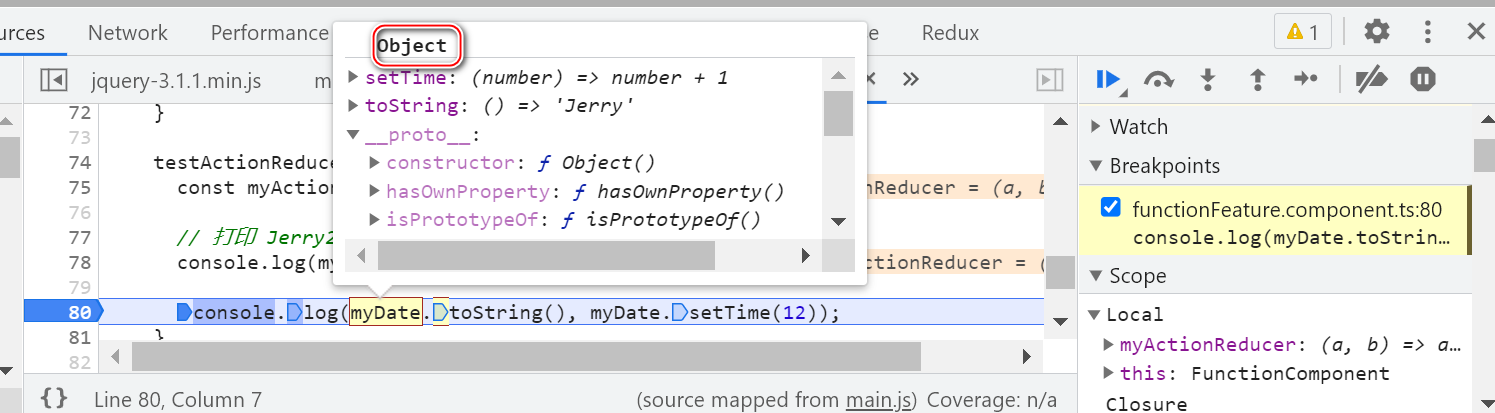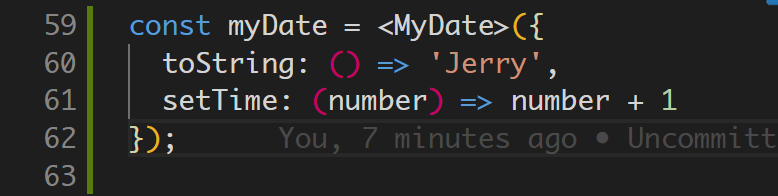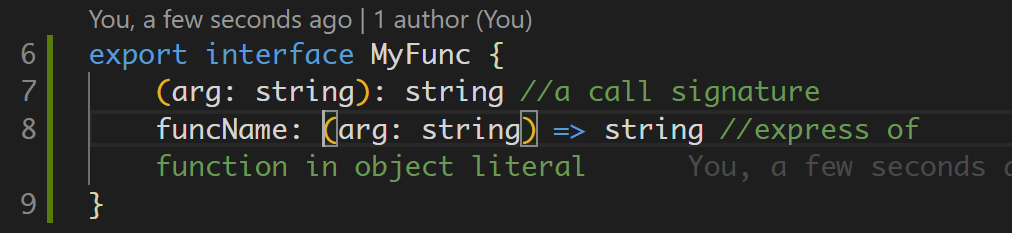# TypeScript 定义函数的几种写法## 写法1 - 使用 function 关键字

``````function greeter(fn: (a: string) => void) {
fn("Hello, World");
}

function printToConsole(s: string) {
console.log(s);
}

greeter(printToConsole);``````
(a: string) => void

``type GreetFunction = (a: string) => void;``

### Call signatures

``````type DescribableFunction = {
description: string;
(someArg: number): boolean;
};

function doSomething(fn: DescribableFunction) {
console.log(fn.description + " returned " + fn(6));
}

const fn = (a: number) => a > 10;

fn.description = 'Jerry';``````

``````type DescribableFunction = {
description: string;
(someArg: number): boolean;
};
const fn = <DescribableFunction>({
description: 'Jerry'
});

const fn23 = Object.assign(
function (number:number) { return number > 1 },
fn
);
function doSomething(fn: DescribableFunction) {
console.log(fn.description + " returned " + fn(6));
}``````

## Construct signatures

``````type SomeConstructor = {
new (s: string): SomeObject;
};
function fn(ctor: SomeConstructor) {
return new ctor("hello");
}``````

## Method Signatures

``````interface Date {
toString(): string;
setTime(time: number): number;
// ...
}````````````const myDate = <MyDate>({
toString: () => 'Jerry',
setTime: (number) => number + 1
});``````

## Function Type Literals

Function Type Literals 是另一种声明函数类型的方法。 它们通常用于高阶函数的签名，即接受函数作为参数或返回函数的函数：

``````interface Array<T> {
sort(compareFn?: (a: T, b: T) => number): this;
// ...
}
``````

``````type FunctionType2 = (string, number) => number;
// (string: any, number: any) => number``````## Object Type Literals with Call or Construct Signatures

``````interface RegExpConstructor {
// Call signatures
(pattern: RegExp): RegExp;
(pattern: string, flags?: string): RegExp;

// ...
}``````

``````interface RegExpConstructor {
// Call signatures
(pattern: RegExp): RegExp;
(pattern: string, flags?: string): RegExp;

// Construct signatures
new (pattern: RegExp): RegExp;
new (pattern: string, flags?: string): RegExp;

// ...
}``````
``````// Using the call signature
const digitsPattern1 = RegExp("^\\d+\$");

// Using the construct signature
const digitsPattern2 = new RegExp("^\\d+\$");``````